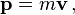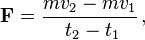# Momentum facts for kids

Kids Encyclopedia Facts
(Redirected from Conservation of momentum)

Momentum can be considered the "power" when an object is moving, meaning how much force it can have on another object. For example, a bowling ball (large mass) pushed very slowly (low velocity) can hit a glass door and not break it, while a baseball (small mass) can be thrown fast (high velocity) and break the same window. The baseball has a larger momentum than the bowling ball. Because momentum is the product of the mass and the velocity of an object, that both mass and velocity affect the momentum of an object. As shown, an object with a large mass and low velocity can have the same momentum as an object with a small mass and large velocity. A bullet is another example where the momentum is very-very high, due to the extraordinary velocity. Another beautiful example where very low-velocities cause greater momentum is the push of Indian subcontinent towards the rest of Asia, causing serious damages, such as earth quakes in the portions of himalayas. In this example, the subcontinent is moving as slow as few inches per year but the mass (imagine weight of India) of Indian-subcontinent is very high.

As defined by Newton, momentum of an object (p) is the product of the mass (m) and velocity (v) of the object. It is a vector quantity, which has both direction and magnitude. Its unit is kg m/s (kilogram metre per second) or N s (newton second). Momentum is sometimes referred to as linear momentum which is different from its related subject angular momentum.

Momentum is a conserved object, meaning that the total initial momentum of a system must be equal to the total final momentum of a system. Total amount of momentum remains unchanged.

## Formula

In Newtonian physics, the usual symbol for momentum is the letter p ; so this can be written$\mathbf{p}= m \mathbf{v}\,,$

where p is the momentum, m is the mass and v is the velocity
If we apply Newton's 2nd Law, we can derive$\mathbf{F}= {mv_2-mv_1\over\ {t_2-t_1}} \,,$

The meaning is that the net force on an object is equal to the rate of change in momentum of the object.

In order to use this equation in special relativity, m has to change with speed. That is sometimes called the "relativistic mass" of the object. (Scientists who work with special relativity use other equations instead.)

## Impulse

Impulse is the change in momentum caused by a new force: this force will increase or decrease the momentum depending on the direction of the force; towards or away from the object that was moving before. If the new force (N) is going in the direction of the momentum of the object (x), the momentum of x will increase; therefore if N is going towards object x in the opposite direction, x will slow down and its momentum will decrease.

## Law of conservation of momentum

In understanding conservation of momentum, the direction of the momentum is important. Momentum in a system is added up using vector addition. Under the rules of vector addition, adding a certain amount of momentum together with the same amount of momentum going in the opposite direction gives a total momentum of zero.

For instance, when a gun is fired, a small mass (the bullet) moves at a high speed in one direction. A larger mass (the gun) moves in the opposite direction at a much slower speed. The momentum of the bullet and the momentum of the gun are exactly equal in size but opposite in direction. Using vector addition to add the momentum of the bullet to the momentum of the gun (equal in size but opposite in direction) gives a total system momentum of zero. The momentum of the gun-bullet system has been conserved.

A collision also shows conservation of momentum: if a car (1000 kg) is going right at 8 m/s, and a truck (6000 kg) is going left at 2 m/s, the car and truck will be moving left after the collision. This exercise shows why:
Momentum = Mass x Velocity
The car's momentum: 1000 kg x 8 m/s = 8000kgm/s (Going right)
The truck's momentum: 6000 kg x -2 m/s = -12000kgm/s (Going left)
This means their total momentum is -4000kgm/s. (Going left)

## Images for kidsMomentum Facts for Kids. Kiddle Encyclopedia.